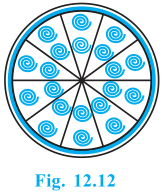# A brooch is made with silver wire in the form of a circle with diameter 35 mm. The wire is also used in making 5 diameters which divide the circle into 10 equal sectors as shown in Fig.. Find: (i) the total length of the silver wire required. (ii) the area of each sector of the brooch.(i) Total length of silver wire required=circumference of broach+5diameter

=5*35*mm*πd

=175+22/7*35

=175+110

=185mm

(ii) Area of each sector=1/10*Area of circle

=1/10*π*r2

=1/10*22/7*35/2*35*2

=11*35/4

=385/4mm2